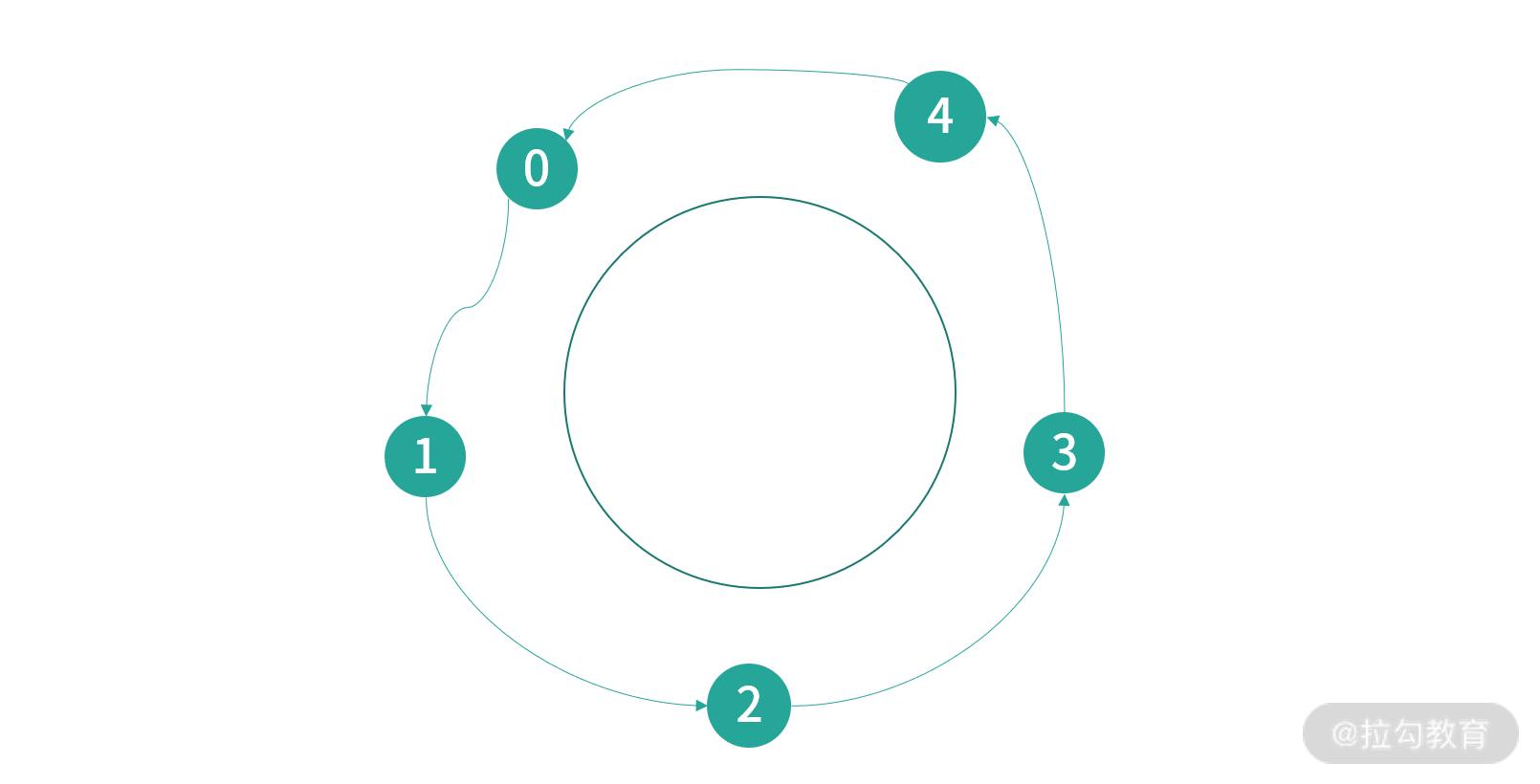21 哲学家就餐问题：什么情况下会触发饥饿和死锁？

哲学家就餐问题问题的抽象

static class Philosopher implements Runnable {

private static Philosopher[] philosophers;

static {

philosophers = new Philosopher;

}

}

private static Integer[] forks;

private static Philosopher[] philosophers;

static {

for(int i = 0; i < 5; i++) {

philosophers[i] = new Philosopher(i);

forks[i] = -1;

}

}

forks[i]的值等于 x，相当于编号为i的叉子被编号为 x 的哲学家拿起；如果等于-1，那么叉子目前放在桌子上。

private static int LEFT(int i) {

return i == 0 ? 4 : i-1;

}

if(forks[LEFT(id)] == id && forks[id] == id) {

// 可以吃面

}

void _take(int i) throws InterruptedException {

forks[i] = id;

}

void _put(int i){

if(forks[i] == id)

forks[i] = -1;

}

_take函数之所以会等待 10ms，是因为哲学家就餐问题的实际意义，是 I/O 处理的场景，拿起叉子好比读取磁盘，需要有一等的时间开销，这样思考才有意义

enum PHIS {

THINKING,

HUNGRY,

EATING

}

void think() throws InterruptedException {

System.out.println(String.format("Philosopher %d thinking...", id));

this.state = PHIS.HUNGRY;

void eat() throws InterruptedException {

synchronized (forks) {

if(forks[LEFT(id)] == id && forks[id] == id) {

this.state = PHIS.EATING;

} else {

return;

}

}

}

while(true){

think();

_take(LEFT(id));

_take(id);

eat();

_put(LEFT(id));

_put(id);

}

void _take(id){

while(forks[id] != -1) {  Thread.yield();  }

}

_take可以考虑阻塞，直到哲学家得到叉子。上面程序我们还没有进行并发控制，会发生竞争条件。 顺着这个思路，就可以想到加入并发控制，代码如下：

while(true){

think();

synchronized(fork[LEFT(id)]) {

_take(LEFT(id));

synchronized(fork[id]) {

_take(id);

}

}

eat();

synchronized(fork[LEFT(id)]) {

_put(LEFT(id));

synchronized(fork[id]) {

_put(id);

}

}

}

• 第 0 个哲学家获得叉子 4，接下来请求叉子 0；
• 第 1 个哲学家获得叉子 0，接下来请求叉子 1；
• 第 2 个哲学家获得叉子 1，接下来请求叉子 2；
• 第 3 个哲学家获得叉子 2，接下来请求叉子 3；
• 第 4 个哲学家获得叉子 3，接下来请求叉子 4。1. 资源存在互斥逻辑：每次只有一个线程可以抢占到资源。这里是哲学家抢占叉子。
2. 持有等待：这里哲学家会一直等待拿到叉子。
3. 禁止抢占：如果拿不到资源一直会处于等待状态，而不会释放已经拥有的资源
4. 循环等待：这里哲学家们会循环等待彼此的叉子。

var lock = new ReentrantLock();

lock.tryLock(5, TimeUnit.SECONDS);

Java 提供的这个能力是拿不到锁，就报异常，并可以依据这个能力开发释放已获得资源的能力。

解决方案

while(true){

synchronized(someLock) {

think();

_take(LEFT(id));

_take(id);

eat();

_put(LEFT(id));

_put(id);

}

}

while(true){

think();

synchronized(someLock) {

_takeForks();

}

eat();

synchronized(someLock) {

_puts();

}

}

void _takeForks(){

if( forks[LEFT(id)] == -1 && forks[id] == -1 ) {

forks[LEFT(id)] = id;

forks[id] = id;

}

}

void _puts(){

if(forks[LEFT(id)] == id)

forks[LEFT(id)] = -1;

if(forks[id] == id)

forks[id] = -1;

}

思考和最终方案

void _transfer(int fork, int philosopher) {

forks[fork] = philosopher;

dirty[fork] = false;

}

void take(int i) throws InterruptedException {

synchronized (forks[i]) {

if(forks[i] == -1) {

_take(id);

} else {

Philosopher other = philosophers[forks[i]];

if(other.state != PHIS.EATING && dirty[i]) {

other._transfer(i, forks[i]);

}

}

}

}

void _take(int i) throws InterruptedException {

forks[i] = id;

}

package test;

import java.util.Arrays;

import java.util.concurrent.ExecutorService;

import java.util.concurrent.Executors;

import java.util.concurrent.TimeUnit;

import java.util.concurrent.atomic.AtomicInteger;

import java.util.concurrent.locks.ReentrantLock;

import java.util.concurrent.locks.StampedLock;

public class DiningPhilosophers {

enum PHIS {

THINKING,

HUNGRY,

EATING

}

static class Philosopher implements Runnable {

private static Philosopher[] philosophers;

private static Integer[] forks;

private static boolean[] dirty;

private PHIS state = PHIS.THINKING;

static {

philosophers = new Philosopher;

forks = new Integer;

dirty = new boolean;

for(int i = 0; i < 5; i++) {

philosophers[i] = new Philosopher(i);

forks[i] = -1;

dirty[i] = true;

}

}

private static int LEFT(int i) {

return i == 0 ? 4 : i-1;

}

public Philosopher(int id) {

this.id = id;

}

private int id;

void think() throws InterruptedException {

System.out.println(String.format("Philosopher %d thinking...", id));

this.state = PHIS.HUNGRY;

}

System.out.println(Arrays.toString(forks));

//System.out.println(Arrays.toString(dirty));

if(forks[LEFT(id)] == id && forks[id] == id) {

this.state = PHIS.EATING;

} else {

return;

}

}

System.out.println(String.format("Philosopher %d eating...", id));

synchronized (forks) {

dirty[LEFT(id)] = true;

dirty[id] = true;

}

var lock = new ReentrantLock();

lock.tryLock(5, TimeUnit.SECONDS);

state = PHIS.THINKING;

}

void _take(int i) throws InterruptedException {

forks[i] = id;

}

void _transfer(int fork, int philosopher) {

forks[fork] = philosopher;

dirty[fork] = false;

}

void _putdown(int i) throws InterruptedException {

forks[i] = -1;

}

void take(int i) throws InterruptedException {

synchronized (forks[i]) {

if(forks[i] == -1) {

_take(id);

} else {

Philosopher other = philosophers[forks[i]];

if(other.state != PHIS.EATING && dirty[i]) {

other._transfer(i, forks[i]);

}

}

}

}

void takeForks() throws InterruptedException {

take(LEFT(id));

take(id);

}

@Override

public void run() {

try {

while(true) {

think();

while (state == PHIS.HUNGRY) {

takeForks();

System.out.println("here--" + Math.random());

eat();

}

}

} catch (InterruptedException e) {

e.printStackTrace();

}

}

}

public static void main(String[] args) {

for(int i = 0; i < 5; i++) {